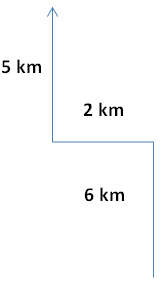# Mini Mock Test SBI Clerk 2018 - Reasoning

(Direction: 1-2) Study the following arrangement carefully and answers the questions.
M 4 P A 3 % R 5 # E J 2 \$ D F 1 U H B @ 9 T W I 8 K N 6 @V 7 Z Q 8 P D

## Question 1.

Is all the symbols in the arrangement are dropped, which of the following will be the 16th from the left end?
1) U
2) 1
3) @
4) 9
5) B

## Question 2.

Which of the following is the eight to the right of the twentieth from the right end of the above arrangement?
1) @
2) 7
3) 6
4) 8
5) J

## Question 3.

Vimal ranks twelfth in a class of 45 students. What is his rank from the last?
1) 29
2) 38
3) 34
4) 36
5) 32

(Direction: 4-5) understand the code language and give the answers of below questions.
In a certain code language ‘ ja na da’ means ‘what you want’ , ‘ na pa ka’ means ‘they want food’ and ‘ ka ho ni’ means ‘ food and water’.

## Question 4.

What does ‘da’ mean in that code language?
1) What or you
2) Want
3) What
4) You
5) None of these

## Question 5.

What does ‘na’ mean in that code language?
1) Food
2) Want
3) You
4) What
5) Water

(Direction: 6-8) To solve the questions, use the meaning of each symbol and follow the directions.
A @ B means ‘A is smaller than B’
A # B means ‘A is either smaller than or equal to B’
A \$ B means ‘A is greater than B’
A * B means ‘A is either greater than or equal to B’
A % B means ‘A is neither greater than nor smaller than B’

## Question 6.

Statement:
H#Q, Q@F, L\$F
Conclusion:
1) L\$H
2) H#F
1) Only conclusion 1 follows
2) Only conclusion 2 follows
3) Either conclusion 1 or conclusion 2 follows
4) Neither conclusion 1 follows nor conclusion 2 follows
5) Both the conclusions to follow

## Question 7.

Statement:
J\$T, T@V, V#M
Conclusion:
1) T#M
2) J%M
1) Only conclusion 1 follows
2) Only conclusion 2 follows
3) Either conclusion 1 or conclusion 2 follows
4) Neither conclusion 1 follows nor conclusion 2 follows
5) Both the conclusions to follow

## Question 8.

Statement:
U#D, D@R, R%T
Conclusion:
1) U@R
2) T\$D
1) Only conclusion 1 follows
2) Only conclusion 2 follows
3) Either conclusion 1 or conclusion 2 follows
4) Neither conclusion 1 follows nor does conclusion 2 follow
5) Both the conclusions to follow

## Question 9.

After walking 5 km Virat turned right and covered a distance of 2 km, then turned left and covered a distance of 5 km. In the end, Virat was moving towards the north. In which direction did Virat started the journey?
1) North
2) South
3) East
4) West
5) Southwest

## Question 10.

Pointing to a woman, a man said’ “her husband’s mother is the wife of my father’s only son.” How is that man related to the woman?
1) Grandfather
2) Father in law
3) None of these
4) Cannot be determined
5) Husband

### Ans 1.  B

M 4 P A 3 R 5 E J 2 D F 1 U H B 9 T W I 8 K N 6 V 7 Z Q 8 P D
Count 16th from the left end= B

### Ans 2. @

When the direction is same, just deduct the small one from big
(20-8)th = 12th from the right end

### Ans 3. 34

Virat’s rank from the last
Total students = Rank from the first + rank form the last – 1
45 = 12 + rank from the last – 1
Rank from the last = 34

H ≤ Q< F < L

T < V ≤ M

U ≤ D < R = T

### Ans 9. South### Ans 10. Father in law

Man’s father’s only son = man himself
Women’s husband’s mother = wife of the man
So, man = Father of the woman's husband
Woman = Wife of man’s son#### What's trending in BankExamsToday

Smart Prep Kit for Banking Exams by Ramandeep Singh - Download here